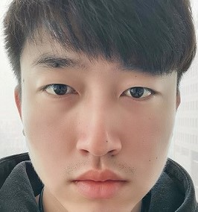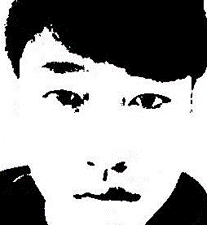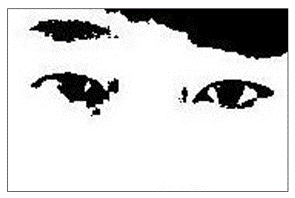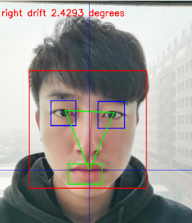A Fatigue Driving Detection Algorithm Based on Support Vector Machine

A Fatigue Driving Detection Algorithm Based on Support Vector Machine
Department of Mathematics, College of Science, Changchun University of Science and Technology, Changchun 130022, China

Corresponding Author Email:
2017100008@mails.cust.edu.cn
Page:
87-92
|
DOI:
https://doi.org/10.18280/rces.060404
12 November 2019
|
Accepted:
17 December 2019
|
Published:
30 December 2019
| Citation

OPEN ACCESS

Abstract:

This paper adopts an algorithm that combines the improved Support Vector Machine (SVM) with facial feature extraction to detect fatigue driving. First, the image is processed to separate the skin color regions in the YCbCr space; second, in the facial area, the positions of the eyes and mouth are roughly located, and then the positions of the eyes are accurately located and the feature parameters of the eyes such as the degree of The Closure of Eye (TCOE) is extracted; third, the geometrical connection lines between the five facial organs are used to extract the feature parameters of the posture of the head, then the extracted parameters and the improved SVM are combined to make classification, thereby judging the degree of fatigue driving of the drivers.

Keywords:

support vector machine (SVM), positioning, feature parameters, degree of fatigue driving

1. Introduction

Fatigue driving is one of the main causes of road traffic accidents. According to statistics, the proportion of traffic accidents caused by fatigue driving accounts for about 30% of all traffic accidents each year . It has caused huge losses to people's lives and property, therefore, if the fatigue state of the driver can be found through technical means and effective warnings could be issued accordingly, the occurrence of traffic accidents can be effectively reduced.

Existing studies on fatigue driving detection are mainly divided into the following three categories: first, the biomedicine-based detection method, which is proposed by Balasubramanian and Adalarasu  in 2007 in their research which determines the onset of fatigue by analyzing biological signals, e.g., the pressure, breathing rate and oxygen debt can be used to estimate the onset of fatigue and the recovery time. Second, the information fusion-based detection method, which is proposed by Wang et al.  in their research that combines EEG recognition with the operating features of the vehicle to detect driver fatigue. Third, the machine vision-based detection method, which is proposed by Devi and Bajaj  in their research in which a system that uses facial image analysis to warn drivers of fatigue and prevent traffic accidents was constructed.

Among the three methods, the biomedicine-based detection method has higher requirements on equipment, and it will affect driving due to the driver's contact with the device, which is not conducive to real-time detection. The information fusion-based detection method involves many aspects, the process is complex and it takes a long time, which is not suitable for practical application. The machine vision-based detection is an indirect detection method, which does not need to contact the human body, and it won’t affect driving; the detection process is simple, and it can rapidly and accurately determine the fatigue state of the driver. Therefore, this paper adopts the machine vision-based detection method for the research.

2. A Fatigue Driving Detection Model Based on SVM

The data collected in this paper is a video clip of the driver during simulated driving recorded by a camera, the video data was subject to framing processing, and the features of the eye status and head postures of the driver were extracted from each frame of the video and taken as the feature parameters of fatigue driving; at last, the improved SVM was applied to judge fatigue driving, and the specific process is shown in Figure 1.

tu_pian_1.pngFigure 1. Fatigue driving judgment process

3. Feature Extraction

3.1 Face detection

Face detection is to detect the position and size of human face in a picture, thereby narrowing down the search area of subsequent work and reducing the amount of calculation. First, the histogram equalization was used to preprocess the image. Histogram equalization is a commonly used method for image enhancement, it can effectively enhance the contrast between the target and the background in the image. Figure 2 shows the effect of histogram equalization.

qqtu_pian_20200307130321.png(a) The original image       (b) The processed image

Figure 2. Histogram equalization

Compared with the complex natural background in the driving cab, the color information of the human face is quite different, so this difference can be used to distinguish the skin color region from the background region . This paper chose to perform it in the Ycbcr color space, so that face detection could be performed better and faster.

This paper used the AdaBoost algorithm based on Haar-like feature rectangle for face detection. Adaboost is an iterative algorithm, its core idea is to train different weak classifiers for the same training set, and then combine these weak classifiers to form a strong classifier . Haar-like features can be used to represent grayscale changes in local areas of the image  and enhance face features, therefore, some of the human face features can be described and represented by Haar-like features, then, the cascade classifier in the AdaBoost algorithm can be used to select the most useful features from the huge amount of rectangular features to construct the final strong classifier.

3.2 Eye feature extraction

3.2.1 Eye positioning

Because the greyscale features of the human eye areas are significantly different from other parts of the human face, it is easy to obtain the approximate positions of the eyes using greyscale integral projection. The idea of the greyscale integral projection algorithm is to accumulate the greyscale values of the pixels in the horizontal and vertical directions of the binary image or the greyscale image, and then obtain the cumulative pixel greyscale curves of the two directions, respectively ; after that, according to the peak and trough distributions in the two curves and the prior knowledge of human face features, the positions of the human eyes can be determined. First, the image was subject to binarization, and the processing result is shown as Figure 3.

According to the prior knowledge of the proportion of human face (the human face can be vertically divided into three equal parts according to the width of the forehead, and horizontally divided into five equal parts according to the length of the eye), we can roughly decide the positions of the human eyes to be between about one-third and two-thirds of the face in the vertical direction, and between about two-fifths and four-fifths of the face in the horizontal direction. As shown in Figure 4.

3a.png(a) The original image

3b.png(b) The processed image

Figure 3. Binarization

Then the segmented regions were subject to horizontal and vertical integral projection to obtain the integral projection curves.

The vertical greyscale integral projection function is as follows:

$p(x)=\sum_{y=1}^{N} f(x, y)$        $x=1,2, \ldots, M$          (1)

The horizontal greyscale integral projection function is as follows:

$p(y)=\sum_{x=1}^{M} f(x, y)$          $y=1,2, \dots, N$          (2)

where, p(x) is the sum of vertical integration, p(y) is the sum of horizontal integration, f(x,y) is the greyscale value of pixel (x,y), M is the width of the image to be detected, and N is the height of the image to be detected. Figure 5 is the curve obtained by performing vertical integral projection on the extracted face region. Figure 6 is the curve obtained by performing horizontal integral projection on the extracted face region.

4.pngFigure 4. The approximate region extracted

5.pngFigure 5. Vertical integral projection curve

6.pngFigure 6. Horizontal integral projection curve

By observing the approximate region extracted from the face image above and its integral projection curves, we can see that the eyebrows are black areas closer to the eyes, therefore, in the horizontal integral projection, the first minimum point and the second minimum point correspond to the y-axis values of the center of the eyebrows and the center of the eyes, respectively. In the vertical direction, the left and right eyes are symmetrical, and the eyebrows and the eyes are on the same vertical line, then in the vertical integral projection, the first minimum point and the second minimum point correspond to the x-axis values of the left eye and the right eye, respectively. In this way, the approximate coordinates of the regions where the centers of the human eyes are located can be obtained.

Since when applying greyscale integral projection method to determine the positions of the human eyes, we can only get the approximate regions of the eyes and the binary image will be disturbed by factors such as light, hair, and eyebrows, etc., therefore, based on greyscale integral projection, this paper adopts the Hough transform detection algorithm to accurately locate the eyes.

Under normal conditions, the pupils of human eyes are generally considered to be precise circles, so if we can find the centers of the two circles in the face image, then we can accurately locate the eyes. The Hough transform algorithm is often used to describe the shape of the boundary of a region, and it is not easily affected by noise or edge discontinuities . Therefore, for pupil contour detection, the Hough transform algorithm is a very effective method. First, the Canny operator is used to detect the edge of the image, then after the contour of the pupils has been detected, Hough transform can be used to search for the circles in the detection region.

The basic idea of Hough transform is to map the spatial domain of the detection image to the parameter space, and describe the edge curve in the image with a certain parameter form that most of the boundary points meet; then through the methods of voting and setting up accumulator, it simplifies the complex problem in the image space to local peak detection problems. The specific process is:

A: First, according to the previously obtained rectangular regions of the left and right eyes, the edge detection is performed, at this time, the Canny operator is used to calculate within the regions;

B: After completing the edge detection of the image, the image is subject to binarization, in this way, the radius of the pupil circle can be determined;

C: Next, the Hough matrix needs to be initialized. First, the accumulator array M is initialized, and the pupil circle parameter lists are all set to 0;

D: Then, draw circles with a radius of r in the rectangular regions of the left and right eyes, detect their edge, and obtain the coordinates of the boundary points, after that, we can obtain the value of $M\left(x_{i}, y_{j}, r\right)$  according to the equation of the circle.

E: Finally, the maximum value of the accumulator in M needs to be calculated, that is, to obtain the coordinates of the center points of the pupil circles.

3.2.2 Eye feature extraction

TCOE (The closure of eye) is measured by the height of the eyes  or the width-height ratio of the eyes, through a large number of experiments we can know that, when the human eyes are completely closed or fully opened, the eyes’ width-height ratio is stable within a small range, and it is less affected by other factors , therefore, within the region of the eyes extracted previously, the height and width of the eyes are calculated by the grayscale integral projection, and then the eyes’ width-height ratio is used to determine the TCOE of the eyes.

As shown in Figure 7: the trough area of the horizontal integral projection map corresponds to the vertical position of the eyes, and the width H of the trough is the height of the eyes. As shown in Figure 8: the trough area of the vertical integral projection map corresponds to the horizontal position of the eyes, and the width W of the trough is the width of the eyes.

7.pngFigure 7. Horizontal integral projection

8.pngFigure 8. Vertical integral projection

Obtain the width W and height H of the human eyes, by calculating the width-height ratio of the eyes we can determine the TCOE, assume TCOE is λ, then there is:

$\lambda=\frac{H}{W}$                                                   (3)

Because the size and shape of the eyes of different drivers are different, for each driver, the width-height ratio λ of the eyes when the driver was in a sober status was collected first, then the width-height ratio λ’ of the eyes when the driver was driving was collected later, then for the TCOE, there is:

$T C O E=\frac{\lambda^{\prime}}{\lambda}$                                          (4)

This paper set the threshold of TCOE to be 50%, that is, when TCOE exceeds 50%, it’s considered that the driver’s eyes are in a closed state, namely:

$T C O E=\left\{\begin{array}{ll} \text { eyes open } & \text { if } \frac{\lambda^{\prime}}{\lambda}>50 \% \\ \text { eyes closed } & \text { if } \frac{\lambda^{\prime}}{\lambda} \leq 50 \% \end{array}\right.$       (5)

Blink frequency refers to the number of times the driver blinks within per unit time, and the driver's blink frequency can reflect the degree of fatigue of the driver to a certain extent. Assume in the image of previous frame, the human eyes’ $\frac{\lambda^{\prime}}{\lambda}>50 \%$ , if in the current frame, the human eyes’ $\frac{\lambda^{\prime}}{\lambda} \leq 50 \%$ , then it’s considered to be a blink.

The driver’s head posture can be used as an important parameter to determine whether the driver is fatigued or not. This paper estimates the head posture by calculating the angle of the facial feature triangle, which is mainly based on the geometry of the face. Under certain conditions, it is believed that the face posture will change with the position deviation of the facial feature triangle. First, the greyscale integral projection algorithm was used to determine the position of the mouth, then with the eyes and the mouth as the three vertices, a feature triangle was constructed as shown in Figure 9. At this time, to roughly estimate the face posture parameters, we only need the coordinates of the three vertices of the feature triangle .

9.pngFigure 9. Feature triangle construction

The head posture estimation is mainly based on the angle of the head's deflection relative to the three coordinate axes, that is, the angle of the head's deflection relative to the z-axis is called the turning angle; the angle of the head's deflection relative to the x-axis is called the nodding angle, and the angle of the head's deflection relative to the y-axis is called the shaking angle [13-14]. Because the driver’s head posture does not change much during the driving process, the rotation of the head relative to the X, Y, and Z axes is within a certain range. Figures 10(a) to 10(d) are pictures for testing head pose estimation. Table 1 is the result of head pose estimation on the picture in Figure 10.

10.pngFigure 10 Test images

Table 1. Correspondence between feature triangle angles and head posture feature parameters

 Images Angles of the feature triangle Head posture feature parameters ÐA ÐB ÐC Turning head Shaking head Nodding head a 50 65 65 0 1 1 b 42 66 72 41 4 3 c 46 69 65 17 0 12 d 47 69 64 2 22 2
4. Fatigue Driving Judgment Method Based on Improved SVM

4.1 Membership function

The key of the fuzzy system is the selection of the membership function. The membership function is a reflection of the importance of the training samples in the training. This paper mainly uses the distance between the sample and the class center to measure the membership degree. Since the relationship between the membership degree of the actual sample and its distance from the class center is not a simple linear relationship, therefore, the S-type function-based membership function was adopted in the following form:

$\mu\left(d_{i} ; a, b, c\right)=\left\{\begin{array}{cc} 1 & d_{i} \leq a \\ 1-2\left[\left(d_{i}-a\right) /(c-a)\right]^{2} & a \leq d_{i} \leq b \\ 2\left[\left(d_{i}-c\right) /(c-a)\right]^{2} & b \leq d_{i} \leq c \\ 0 & d_{i} \geq c \end{array}\right.$   (6)

where, diis the distance between the sample and the center of the class, parameters a, b, and c are predefined parameters, and there is $b=\frac{a+c}{2}$ ; when di=b, there is μ(di;a,b,c)=0.5.

4.2 Fuzzy SVM

When applying fuzzy SVM for classification, firstly, the fuzzy membership μ(xi) should be introduced to the training set, so that the training set becomes a fuzzy training set, and the expression form of the fuzzy training set changes to:

$\left\{\left(\mathrm{x}_{1}, \mathrm{y}_{1}, \mu\left(x_{1}\right)\right), \ldots\left(\mathrm{x}_{\mathrm{i}}, \mathrm{y}_{\mathrm{i}}, \mu\left(x_{i}\right)\right), \ldots\left(\mathrm{x}_{\mathrm{n}}, \mathrm{y}_{\mathrm{n}}, \mu\left(x_{n}\right)\right)\right\}$

where, $x_{i} \in R^{n}$ is the feature of each sample, $y_{i} \in\{-1,1\}$ is the class identification, and the membership is $0<\mu\left(x_{i}\right) \leq 1$. The corresponding optimization problem is as follows:

$\left\{\begin{array}{c} \min \left(\frac{1}{2}\|w\|^{2}+C \sum_{i=1}^{n} \mu\left(x_{i}\right) \xi_{i}\right) \\ \text { s.t. } \quad y_{i}\left(w^{T} \varphi\left(x_{i}\right)+b\right) \geq 1-\xi_{i} \\ \xi_{i} \geq 0 \end{array}\right.$                   (7)

where, ξi is the relaxation variable, C is the penalty factor, $\phi\left(x_{i}\right)$ is the mapping of training samples from low-dimensional space to high-dimensional space.

The corresponding Lagrange function is as follows:

\begin{aligned} &L(w, b, \xi)=\frac{1}{2}\|w\|^{2}+C \sum_{i=1}^{n} \mu\left(x_{i}\right) \xi_{i}-\\ &\sum_{i=1}^{n} \alpha_{i}\left[y_{i}\left(w \cdot x_{i}+b\right)-1+\xi_{i}\right]-\sum_{i=1}^{n} \beta_{i} \xi_{i} \end{aligned}                (8)

where, αi≥0 and βi≥0 are the Lagrange multipliers.

Take the derivative of w, b, and ξ, respectively, we can obtain:

$\left\{\begin{array}{r} \frac{\partial L}{\partial w}=w-\sum_{i=1}^{n} \alpha_{i} y_{i} x_{i} \\ \frac{\partial L}{\partial b}=-\sum_{i=1}^{n} \alpha_{i} y_{i} \\ \frac{\partial L}{\partial \xi}=\mu\left(x_{i}\right) C-\alpha_{i}-\beta_{i} \end{array}\right.$                             (9)

Let the equations in (9) be equal to zero respectively and introduce them into equations (8) to obtain the dual problem as follows:

$\left\{\begin{array}{c} \max \sum_{i=1}^{n} \alpha_{i}-\frac{1}{2} \sum_{i=1}^{n} \sum_{j=1}^{n} \alpha_{i} \alpha_{j} y_{i} y_{j}\left(x_{i} \cdot x_{j}\right) \\ \text { s.t. } \quad \sum_{i=1}^{n} \alpha_{i} y_{i}=0 \\ 0 \leq \alpha_{i} \leq \mu\left(x_{i}\right) C \quad i=1,2, \ldots, n \end{array}\right.$           (10)

Finally, the corresponding decision function formula is obtained as:

$\begin{array}{c} f(x)=\operatorname{sgn}\left(\sum_{i=1}^{n} y_{i} \alpha_{i}^{*} K\left(x_{i}, x_{j}\right)+b^{*}\right) \\ \text { s.t. } \quad 0 \leq \alpha_{i}^{*} \leq \mu\left(x_{i}\right) C \end{array}$                 (11)

where, K(xi,xj) is the kernel function, which converts the inner product on a high-dimensional space into a simple function calculation on a low-dimensional space. Here we adopt a Gaussian kernel function $K\left(x_{i}, x_{j}\right)=\exp \left(-\frac{\left\|x_{i}-x_{j}\right\|^{2}}{2 \sigma^{2}}\right)$ , σ is the width of the Gaussian distribution.

4.3 Fatigue driving judgment

The eye status and head posture extracted above are taken as parameters and combined with the fuzzy SVM to determine the driving fatigue.

(1) Fuzzy SVM training and testing

This paper took the eye status feature parameters and head posture feature parameters extracted within ten seconds under the fatigue driving condition as the positive sample features; and the same parameters that were extracted under the sober driving condition were taken as the negative sample features. Due the limitation of the experimental conditions, 600 male positive sample data and 400 female positive sample data were extracted from the simulated driving videos of 5 drivers, and 600 male negative samples and 400 female negative samples were taken as a training set, which was used to train the SVM.

(2) Comparison of experimental results

The output parameter of the fatigue driving judgment model is the fatigue driving degree, which is divided into 3 levels, respectively are: level-A (sober), level-B (slight fatigue), and level-C (severe fatigue). Below is a comparison of the experimental results of ordinary SVM and improved SVM.

Three sets of experimental data were extracted to test the results of the ordinary SVM and the improved SVM. The three sets of data were the eye status feature parameters and the head posture feature parameters extracted within ten seconds under the sober status, slight fatigue status and severe fatigue status during the simulated driving, wherein each data set had 100 male data.

Table 2 shows the fatigue driving judgment results of ordinary SVM, and Table 3 shows the fatigue driving judgment results of fuzzy SVM.

The comparison of the two sets of experimental results shows that, in terms of fatigue driving determination, the fuzzy SVM can make better judgement than the traditional SVM.

Table 2. Fatigue driving judgment results of ordinary SVM

 Degree of fatigue 100 male data in each set 50 female data in each set Sober Slight fatigue Severe fatigue Sober Slight fatigue Severe fatigue Sober 87 16 15 45 9 7 Slight fatigue 13 78 3 5 37 3 Severe fatigue 0 6 82 0 4 40 Accuracy 87% 78% 82% 90% 74% 80%

Table 3. Fatigue driving judgment results of fuzzy SVM

 Degree of fatigue 100 male data in each set 50 female data in each set Sober Slight fatigue Severe fatigue Sober Slight fatigue Severe fatigue Sober 92 9 7 46 5 4 Slight fatigue 8 87 2 4 41 1 Severe fatigue 0 4 91 0 4 45 Accuracy 92% 87% 91% 92% 82% 90%
5. Conclusion

This paper conducted an in-depth and detailed research on fatigue driving. By examining the features of the eyes and the head of the driver, it extracted feature data and conducted statistical analysis on the extracted feature data to judge the fatigue status of the driver. The study applied improved SVM to fatigue driving judgement, compared with the traditional PERCLOS criterion based on eye status, the proposed method in this paper added head posture parameters, and it has higher accuracy and application value.

References

 Jiang, S.Y. (2016). Study on the harm of driver's fatigue to safe driving. Auto Time, 2016(11): 33-34. https://doi.org/10.3969/j.issn.1672-9668.2016.11.012

 Balasubramanian, V., Adalarasu, K. (2007). EMG-based analysis of change in muscle activity during simulated driving. Journal of Bodywork and Movement Therapies, 11(2): 151-158. https://doi.org/10.1016/j.jbmt.2006.12.005

 Wang, F., Wang, S.N., Wang, X.H., Peng, Y., Yang, Y.D. (2014). Driving fatigue detection based on EEG recognition and vehicle handling characteristics. Chinese Journal of Scientific Instrument, 35(2): 398-404. https://doi.org/10.3969/j.issn.0254-3087.2014.02.022

 Devi, M.S., Bajaj, P.R. (2010). Fuzzy based driver fatigue detection. In 2010 IEEE International Conference on Systems, Man and Cybernetics, pp. 3139-3144. https://doi.org/10.1109/ICSMC.2010.5641788

 Lal, S.K., Craig, A., Boord, P., Kirkup, L., Nguyen, H. (2003). Development of an algorithm for an EEG-based driver fatigue countermeasure. Journal of safety Research, 34(3): 321-328. https://doi.org/10.1016/S0022-4375(03)00027-6

 Huguenin, R.D. (1988). The concept of risk and behavior models in traffic psychology. Ergonomics, 31(4): 557-569. https://doi.org/10.1080/00140138808966699

 Vural, E., Cetin, M., Ercil, A., Littlewort, G., Bartlett, M., & Movellan, J. (2007). Drowsy driver detection through facial movement analysis. In International Workshop on Human-Computer Interaction, 4796: 6-18. https://doi.org/10.1007/978-3-540-75773-3_2

 Chen, X.Y., Wang, Q., Li, B.L. (2015). Improved hough algorithm for circle detection. Computer Systems & Applications, 24(8): 197-199. https://doi.org/10.3969/j.issn.1003-3254.2015.08.035

 Wang, G.H., Kong, M., He, Y. (2005). Hough transform and its application in information processing. Beijing: Ordnance Industry Press, 2005.

 Jiang, J.G., Liu, Y., Zhan, S., Li, H.L. (2008). Real-time driving fatigue detection in gray image sequence. Journal of Hefei University of Technology (Natural Science), 31(9): 1424-1427, 1442. https://doi.org/10.3969/j.issn.1003-5060.2008.09.019

 Mao, X.W., Jing, W.B., Wang, X.M., Liu, X., Zhang, S.S. (2016). A fatigue driving detection method based on eye state. Journal of Changchun University of Science and Technology, 39(2): 125-130. https://doi.org/10.3969/j.issn.1672-9870.2016.02.027

 Morency, L. P., Whitehill, J., & Movellan, J. (2010). Monocular head pose estimation using generalized adaptive view-based appearance model. Image and Vision Computing, 28(5): 754-761. https://doi.org/10.1016/j.imavis.2009.08.004

 Xiao, R., Wang, J.C., Zhang, F.Y. (2000). Overview of support vector machine theory. Computer Science, 27(3): 1-3. https://doi.org/10.3969/j.issn.1002-137X.2000.03.001

 Shao, H.H. (2003). Support vector machine theory and its application. Automation Panorama, 20(Z1): 90-95. https://doi.org/10.3969/j.issn.1003-0492.2003.z1.022